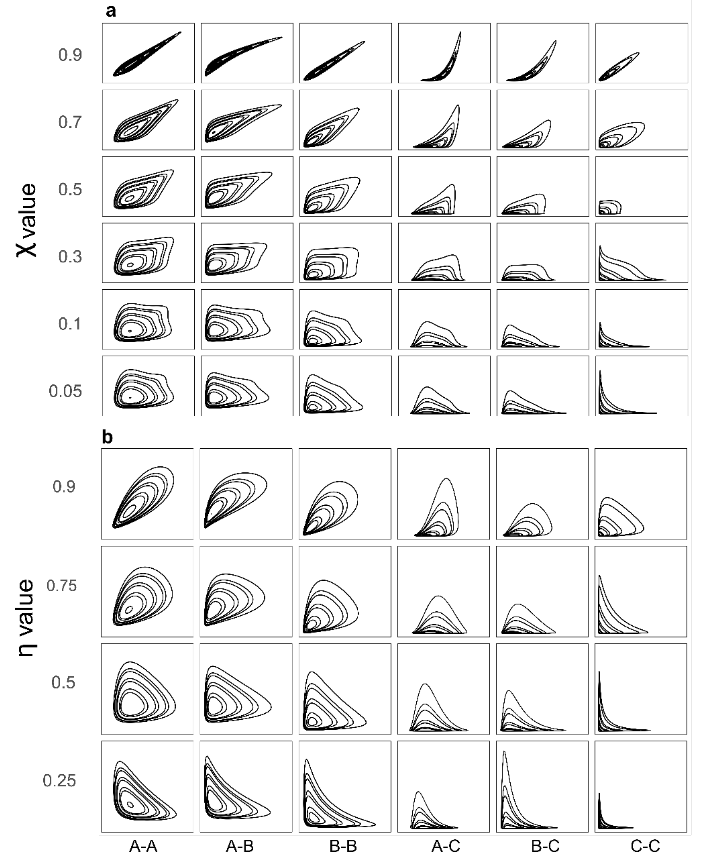# how to use the package

library(mobirep)
#> Loading required package: texmex
#> Loading required package: mvtnorm
#> Loading required package: ggplot2

# Package mobirep

This package is associated with the article “Evaluating the efficacy of bivariate extreme modelling approaches for multi-hazard scenarios” https://nhess.copernicus.org/articles/20/2091/2020/nhess-20-2091-2020.html It includes 6 models, the joint tail KDE model derived from Cooley et al. (2019) https://doi.org/10.1007%2Fs10687-019-00348-0, the conditional extreme model initially developed by Heffernan and Tawn (2004) https://doi.org/10.1111/j.1467-9868.2004.02050.x and four copulae: Normal, Farlie-Gumbel-Morgenstern, Galambos and Gumbel.

## Selection of analogous synthetic datasets from Tilloy et al. (2020)

### 60 bivariate datasets* Import data example bivariate dataset of daily mean temperature (data from E-OBS; Cornes et al., 2018) and number of wildfire per day in Porto district (Portugal)

data(porto)
• Use function Analogsel to identify which synthetic datasets from Tilloy et al. (2020) are analogs of the imput data
AnalogSel(fire01meantemp)

## Model the marginal distributions of the dataset

select extreme threshold for the two margins

tr1=0.9
tr2=0.9

remove NA form the dataset

fire01meantemp=na.omit(fire01meantemp)
u=fire01meantemp

fit a GPD to both margins and create extrapolated time serie (useful to create level curves)

margins=Margins.mod(tr1,tr2,u=fire01meantemp)


## Fit bivariate models to the data

jtres<-JT.KDE.ap(u2=u,pb=0.01,pobj=upobj,beta=100,
vtau=vtau,kk=kk,devplot=F,mar1=uu[,1],mar2=uu[,2],
px=pp[,1],py=pp[,2],interh=interh)

## Create level curve with a given amount of point and compute the density of the curves (for most likely scenario)

jt.dens<-kde(u,gridsize = 200)
ltlo<-digit.curves.p(start=jtres\$wq0ri[1,], as.matrix(wq0ri), nPoints=98, closed = FALSE)
ltl<-densi.curv.em(jt.dens,ltlo, tl="l", ltl)